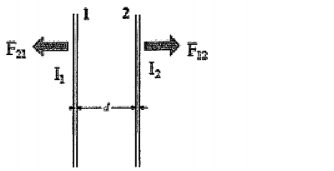# Problem: Two wires are separated by d and carry currents I1 and I2, respectively. However, we don't know yet the direction of the current I1 and I2. What we knnow is that the two wires repel each other. Which of the following false? a. The direction of current I1 and I2 are opposite b. Both F12 and F21 are inversely proportional to the square of the distance  d c. Always F12 = -F21 even if I1 is larger than I2 d. The magnitude of the magnetic field in the area between the two wires is stronger than those in the other regions e. There is no point in the area between the two wires, where the magnetic field is  zero

92% (46 ratings)
###### Problem Details

Two wires are separated by and carry currents I1 and I2, respectively. However, we don't know yet the direction of the current I1 and I2. What we knnow is that the two wires repel each other. Which of the following false?

a. The direction of current I1 and I2 are opposite

b. Both F12 and F21 are inversely proportional to the square of the distance  d

c. Always F12 = -F21 even if I1 is larger than I2

d. The magnitude of the magnetic field in the area between the two wires is stronger than those in the other regions

e. There is no point in the area between the two wires, where the magnetic field is  zeroFrequently Asked Questions

What scientific concept do you need to know in order to solve this problem?

Our tutors have indicated that to solve this problem you will need to apply the Magnetic Force Between Parallel Currents concept. You can view video lessons to learn Magnetic Force Between Parallel Currents. Or if you need more Magnetic Force Between Parallel Currents practice, you can also practice Magnetic Force Between Parallel Currents practice problems.

How long does this problem take to solve?

Our expert Physics tutor, Juan took 5 minutes and 11 seconds to solve this problem. You can follow their steps in the video explanation above.